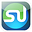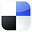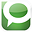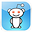# To Find Your Sales Curve

Excel Solver is one of the best and easiest curve-fitting devices in the world, if you know how to use it. The marketing manager will find the curve-fitting capability of the Excel Solver to be the perfect tool to create a predictive sales equation.

In this example we are going to show how to use the Excel Solver to calculate an equation which most closely describes the relationship between sales and number of ads being run. The purpose of this equation is to be able to predict the number of sales based upon the number of ads that will be run.

A marketing manager has collected this following data on the company’s sales vs. the number of ads that were running at different times.

50             6700
55             7500
59             8700
62             8900
75             8800
95             10900
110           11200
125           11400
140           11500
180           12300

Here is an Excel scatter plot of that data:

Click On Image To See a Larger Version

We would like to create an equation from this data that allows us to predict the sales based upon the number of ads currently running.

The first step is to eyeball the data and estimate what general type of curve this graph probably is. In this case it appears to a graph the has a diminishing y value for an increasing x value. A formula for such a curve would have the general form:

Y = A1 + A2 * X**B1

Sales = A1 + A2 * (Number of Ads Running)**B1

We can use the Excel Solver to solve for A1, A2, and B1.
We need to arrange the data in a form that can be input into the Excel Solver as follows:

This table shows the arrangement of data and the calculations. Here we have created an Excel model based upon our model of:

Sales = A1 + A2 * (Number of Ads Running)B1

One example of this formula in action is explained for Cell E16. We are listing the variable that we are solving for (A1, A2, and B1) in cells B3 to B5. In Solver language, these solves that we are changing are called Decision Variables.

We have arbitrarily set our Decision Variables for:

A1 = 100

A2 = 100
B1 = 0.05

We now take the difference between the actual number of sales and the number of sales predicted by our model with our arbitrary settings for the Decision Variables. The square of each difference is taken and then all squares are summed up.

We are trying to find the settings for the Decision Variables that will minimize the sum of the squares of the differences. In other words, we are trying to find A1, A2, and B1 that will minimize the number in cell G13.

Once the Solver has been installed as an add-in (To add-in Solver: File / Options / Add-Ins / Manage / Excel Add-Ins / Go / Solver Add-In), you can access the Solver in Excel 2010 by: Data / Solver.

The following blank Solver dialogue box comes up:

Click On Image To See a Larger Version

The Solver dialogue box has the following 5 parameters that need to be set:

1) The Objective Cell – The is the target cell that we are either trying to maximize, minimize, or achieve a certain value.

2) Whether we want to minimize or maximize the target, or attempt to achieve a certain value in the Objective cell.

3) Decision Variables – A set of variables that will be changed by the Excel Solver in order to optimize the target cell.

4) Constraints – These are the limitations that the problem subjects the Solver to during its calculations

Once again, here is the data table for Solver inputs:

Click On Image To See a Larger Version

Objective:

We are trying to minimize Cell G13, the sum of the square of differences between the actual and predicted sales.

Decision Variables:

We are changing A1, A2, and B1 (cells B3 to B5) to minimize our Objective, Cell G13. The Decision Variables are therefore Cells B3 to B5.

Constraints:
There are none for this curve-fitting operation.

Selection of Solving Method: GRG Nonlinear

The GRG Nonlinear method is used when the equation producing the objective is not linear but is smooth (continuous). Examples of smooth nonlinear functions are:
=1/C1, =Log(C1), and =C1^2

These functions have graphs that are curved (nonlinear), but have no breaks (smooth)

Our sales equation appears to be smooth and non-linear:

Sales = A1 + A2 * (Number of Ads Running)**B1

Here is the completed Solver dialogue box:

Click On Image To See a Larger Version

Here is a close-up of the Solver Objective, Decision Variables, and Contraints:

Click On Image To See a Larger Version

If we now hit the Solve button, we get the following result:

Click On Image To See a Larger Version

Solver has optimized the Decision Variables to minimize the objective function as follows:

A1 = -445,616
A2 = 437,247
B1 = 0.00911

The Objective is minimized to: 2,556,343

We can now create an Excel graph of the Actual Sales vs. the Predicted Sales as follows:

Click On Image To See a Larger Version

Solver calculates that Sales can be predicted from Number of Ads Runing bythe following equation:

Sales = -445616 + 437247 * (Number of Ads Running)**(.00911)

The trickiest part of this problem is the first step; eyeballing the data to determine what kind of graph the data is arranged in. You should take time to evaluate whether you are pursuing calculation of the correct curve type.

Solver Tips

You may notice that if you run this problem through the Solver multiple time, you will get slightly different answers. Each time that you run Solver’s GRG algorithm, it will calculate different values for the Decision Variables. You are trying to find the values for the Decision Variables that minimize the objective function (cell G13) the most.

When the Solver runs the GRG algorithm, it picks a starting point for its calculations. Each time you run the Solver GRG method a slightly different starting point will be picked. That is why different answers will appear during each run. Choose the Decision Variable value that occur during the run which produces the lowest value of the Objective. Keep running the Solver until the objective is not minimized anymore. That should give you the optimal values of the Decision Variables. That was done in the example above.

Initial Solver Settings:

Here are some Solver settings that you want to configure prior to running the Solver for most problems. These settings are found when you click the Options button:

Show Iteration Results: Leave this unchecked. This stops the GRG Solver after each iteration, displaying the result for that iteration. Very rarely is there a reason for doing that.

Use Atomatic Scaling: Leave this box unchecked. You would only use this option if you had reason to believe that inputs of the Solver were measured using different scales.

Assume Non-Negative: Only check this if you are sure that none of the variables can ever be negative. In this case, that is clearly not the case.

Bypass Solver Reports: Leave this box unchecked. There is no advantage to not having Solver reports for each Solver run.

Summary

Excel Solver is an easy-to-use and powerful curve fitting tools that can be used to find predictive sales equations for your company. It will work as long as you have properly determined the correct general curve type in the beginning.

If you would like to create a link to this blog article, here is the link to copy for your convenience: Using Excel Solver to Find Your Sales EquationIf You Like This, Then Share It...Excel Master Series Blog Directory

Statistical Topics and Articles In Each Topic

• Histograms in Excel
• Bar Chart in Excel
• Combinations & Permutations in Excel
• Normal Distribution in Excel
• t-Distribution in Excel
• Binomial Distribution in Excel
• z-Tests in Excel
• t-Tests in Excel
• Hypothesis Tests of Proportion in Excel
• Chi-Square Independence Tests in Excel
• Chi-Square Goodness-Of-Fit Tests in Excel
• F Tests in Excel
• Correlation in Excel
• Pearson Correlation in Excel
• Spearman Correlation in Excel
• Confidence Intervals in Excel
• Simple Linear Regression in Excel
• Multiple Linear Regression in Excel
• Logistic Regression in Excel
• Single-Factor ANOVA in Excel
• Two-Factor ANOVA With Replication in Excel
• Two-Factor ANOVA Without Replication in Excel
• Creating Interactive Graphs of Statistical Distributions in Excel
• Solving Problems With Other Distributions in Excel
• Optimization With Excel Solver
• Chi-Square Population Variance Test in Excel
• Analyzing Data With Pivot Tables
• SEO Functions in Excel
• Time Series Analysis in Excel

1.I dont understand what A1, A2 and B1 mean

2.The sales data is free and gives you thoughts and sales systems to experiment with and use for yourself.sales coaching

3.Thanks for a wonderful share. Your article has proved your hard work and experience you have got in this field. Brilliant .i love it reading. איש יחסי ציבור

4.thanks this is good blog. קורס מכירות

5.NLP is an arrangement of procedures that help you associate with your customers and by utilizing the strategies you can likewise conquer sales hindrances. Here is a rundown of sales tips that can truly help your sales numbers to take off.cold call script

6.How a sales training course, with some extraordinary systems, was created to give every individual from a normal group a compelling procedure and selling achievement.Cold call appointment script

7.Sales training articles have also begun to realize how understanding the competition can provide sales success. This is no surprise, however, as interested customers will be comparing your services and products from the competition.website conversion tools

8.Selling too low and talking to the wrong people in the sales process is another common sales strategy mistake. B2B Data List

9.Relevant information is surfaced for each screen, streamlining process and workflows, with Lightning. Salesforce training in Chennai